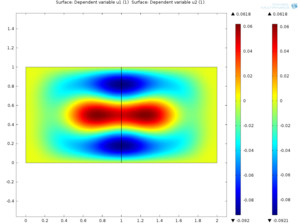# Application ExchangeFilename Size
mb3.mph 174 KB

## Domain decomposition for hyperbolic equations

mikhael balabane, universite paris 13

Domain decomposition for hyperbolic equations
Mikhael Balabane , Université Paris 13
Stephan Savarese, COMSOL France

This model shows how to solve an iterative algorithm using domain decomposition techniques.

Coefficient Form PDE u1 (c4) solves for u1
Coefficient Form PDE u2 (c) solves for u2
Coefficient Form PDE v1 (c2) stores u1 into v1
Coefficient Form PDE v2 (c3) stores u2 into v2
Then compute and iterate as follows:

1. Compute Init U
2. In LOOP > Step1 > Values of Variables not solved for : select Solution : Init U , then Compute
3. In LOOP > Step1 > Values of Variables not solved for : select Solution : LOOP, then Compute as many times as necessary to converge